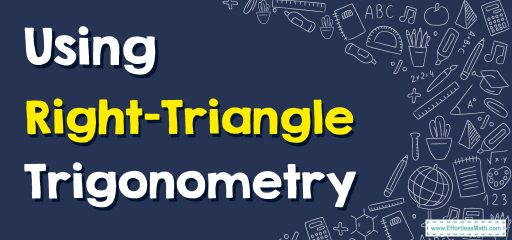# How to Use Right-Triangle Trigonometry

Right-triangle trigonometry is the study of the relationships between the angles and side lengths of a right triangle. The three main trigonometric functions in a right triangle are sine $$(sin)$$, cosine $$(cos)$$, and tangent $$(tan)$$. These functions are defined in terms of the ratios of the sides of a right triangle.## Related Topics

The Absolute Best Book to Ace Trigonometry

## Step-by-step to use right-triangle trigonometry

Here are some examples of how to use right-triangle trigonometry to solve problems:

1. Finding the length of a side: To find the length of a side of a right triangle, you can use one of the trigonometric ratios. For example, if you know the measure of an angle and the length of the opposite side, you can use the sine function to find the length of the hypotenuse.
2. Finding the measure of an angle: To find the measure of an angle of a right triangle, you can use one of the trigonometric ratios. For example, if you know the length of the opposite side and the hypotenuse, you can use the sine function to find the measure of the angle.
3. Solving a word problem: To solve a word problem involving a right triangle, you can use trigonometric ratios to find missing information. For example, if a ladder leans against a wall and makes an angle of $$60$$ degrees with the ground, and the ladder is $$10$$ feet long, you can use the tangent function to find the height of the wall.
4. Trigonometric Identities: You might be required to use trigonometric identities such as $$sin^2(x) + cos^2(x) = 1$$, $$cot(x) = \frac{1}{tan(x)}$$, $$cosec(x) = \frac{1}{sin(x)}$$ and many more to simplify your work and make it more efficient.

It’s important to notice that trigonometry can be used in various fields such as physics, engineering, navigation, and many more.

A good practice is to draw a diagram and label it properly to help you visualize the problem and identify the known and unknown quantities.

The Best Books to Ace the Trigonometry Test

### What people say about "How to Use Right-Triangle Trigonometry - Effortless Math: We Help Students Learn to LOVE Mathematics"?

No one replied yet.

X
51% OFF

Limited time only!

Save Over 51%

SAVE $15 It was$29.99 now it is \$14.99